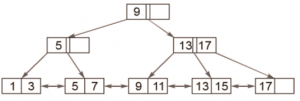Exercise: Questions on B and B+ Trees

Questions on B and B+ Trees : Question 1 :
Consider a B+-tree in which the maximum number of keys in a node is 5. What is the minimum number of keys in any non-root node? (GATE CS 2010)

 1 2  Correct 3 4
Questions on B and B+ Trees : Question 2 :
Which one of the following is a key factor for preferring B-trees to binary search trees for indexing database relations?

 Database relations have a large number of records Database relations are sorted on the primary key B-trees require less memory than binary search trees Data transfer form disks is in blocks.  Correct
Questions on B and B+ Trees : Question 3 :
B+ trees are preferred to binary trees in databases because (GATE CS 2000)

 Disk capacities are greater than memory capacities Disk access is much slower than memory access  Correct Disk data transfer rates are much less than memory data transfer rates Disks are more reliable than memory
Questions on B and B+ Trees : Question 4 :
Which of the following is FALSE about B/B+ tree

 B/B+ trees grow upward while Binary Search Trees grow downward. Time complexity of search operation in B/B+ tree is better than Red Black Trees in general.  Correct Number of child pointers in a B/B+ tree node is always equals to number of keys in it plus one. A B/B+ tree is defined by a term minimum degree. And minimum degree depends on hard disk block size, key and address sizes.
Questions on B and B+ Trees : Question 5 :
A B-tree of order 4 is built from scratch by 10 successive insertions. What is the maximum number of node splitting operations that may take place?

 3 4 5  Correct 6
Questions on B and B+ Trees : Question 6 :
The order of a leaf node in a tree B+ ? is the maximum number of (value, data record pointer) pairs it can hold. Given that the block size is 1K bytes, data record pointer is 7 bytes long, the value field is 9 bytes long and a block pointer is 6 bytes long, what is the order of the leaf node?

 63  Correct 64 67 68
Questions on B and B+ Trees : Question 7 :
The order of an internal node in a B+ tree index is the maximum number of children it can have. Suppose that a child pointer takes 6 bytes, the search field value takes 14 bytes, and the block size is 512 bytes. What is the order of the internal node?

 24 25 26  Correct 27
Questions on B and B+ Trees : Question 8 :

 A B  Correct C D
With reference to the B+ tree index of order 1 shown below, the minimum number of nodes (including the root node) that must be fetched in order to satisfy the following query: Get all records with a search key greater than or equal to 7 and less than 15 is ________